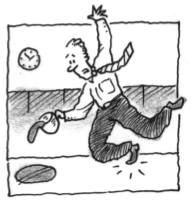### Home > CALC > Chapter 7 > Lesson 7.1.4 > Problem7-40

7-40.

As clumsy Kenny poured his cold coffee on the floor, he noticed that the growing puddle was circular. Let A be the area of a circle with radius $r$ at time $t$. Write an equation that relates $\frac { d A } { d t }$ to $\frac { d r } { d t }$.

$A = πr^2$

Implicitly differentiate both sides of the area function WITH RESPECT TO TIME, $t$.